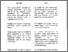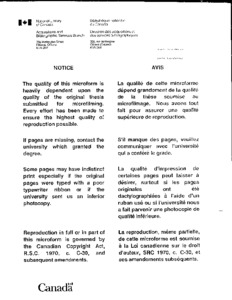Title:

# Connectedness of the attractor of an iterated function system

Sandoghdar, Farid (1995) Connectedness of the attractor of an iterated function system. Masters thesis, Concordia University.Preview
Text (application/pdf)
MM05129.pdf
1MB

## Abstract

We will study the action of a finite family, $\{ F\sb i\} \sbsp {i=1}{m},$ of contractive mappings on a compact subset of a complete metric space. The family $\{ F\sb i\} \sbsp {i=1}{m},$ is known as an iterated function system (IFS). Although many of the results presented here can be generalized to an arbitrary complete metric space, we will restrict ourselves to the metric space ($\IR\sp{n}$, Euclidean distance). Using the notion of Minkowski sausages, we will define a distance function, h (the Hausdorff distance), on the space of all compact subsets of $\IR\sp{n}$ denoted by ${\cal H}(\IR\sp{n})$. The pair (${\cal H}(\IR\sp n), h)$ forms a complete metric space in which we will establish the existence of a unique "point" ${\cal A}$ (compact subset of $\IR\sp n)$ satisfying the equation $\cup \sbsp{i=1}{m}\ F\sb i({\cal A}) = {\cal A}.$ We will explore various characterizations of the compact set ${\cal A}$ which is referred to as the attractor of the IFS $\{ F\sb i\} \sbsp {i=1}{m}$. The topological properties of an attractor depend on the contractive mappings constituting the corresponding IFS. The main purpose of this study is to investigate conditions under which an attractor will have certain connectivity properties. Among these properties will be considered total disconnectedness, connectedness and arcwise connectedness. We will see that in fact, the notions of connectedness and arcwise connectedness coincide in the case of an attractor. Some other topological properties of attractors including property S, local connectedness and semi-local connectedness will also be discussed.

Divisions: Concordia University > Faculty of Arts and Science > Mathematics and Statistics Thesis (Masters) Sandoghdar, Farid ix, 60 leaves : ill. ; 29 cm. Concordia University M.Sc. Mathematics 1995 Tricot, Claude 2833 Concordia University Library 27 Aug 2009 19:21 18 Jan 2018 17:20 http://clues.concordia.ca/search/c?SEARC...
All items in Spectrum are protected by copyright, with all rights reserved. The use of items is governed by Spectrum's terms of access.

Repository Staff Only: item control pageResearch related to the current document (at the CORE website)
Back to top